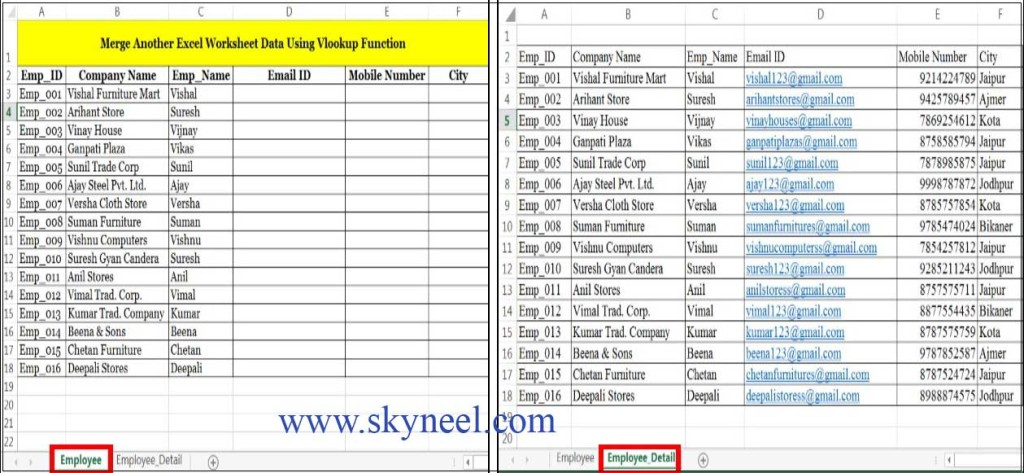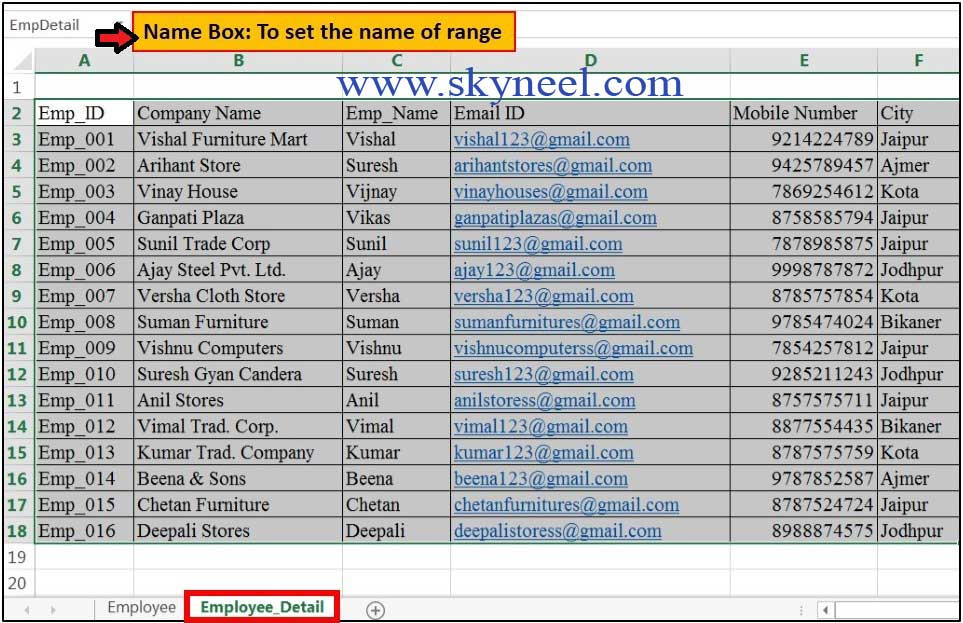# Vlookup merge data from one sheet to another Excel worksheet

Vlookup merge data from one sheet to another Excel worksheet, which provide the facility to easily import one worksheet data into other.

Hi, everyone today we discuss another very important topic which you face during doing job in your company or facing the interview session. How to Vlookup merge data from one sheet to another Excel worksheet? The answer to this query is either you have to use copy and paste method or you can also use use MS Excel’s most popular “Vlookup” function to easily merge or combine another sheet data into different sheet. This function is found in the lookup and reference category of Microsoft Excel So have a look and take few easy steps to easily overcome this kind of problem.

#### Syntax of VLookup merge data from one sheet to another Excel worksheet:

The Microsoft Excel VLookup function search a value in the extreme left column of the table_array and provide the value in the same row which is based on the index_number.

=VLookup (lookup_value, table_array, col_index_num, [range])

Note: Before using the VLookup function, first you need to arrange first column of a table or array in the ascending order, otherwise VLookup function is not able to provide the accurate result.

Lookup_value: The value to be searched in the extreme first column of the table array.
Table_array: Two or more columns of data. Give cell reference of a range / range name.
Col_index_num: The column index_number in the table from which the searched value must be
returned.

If col_index_num of returns 1 the value in the first column in table_array.
If col_index_num is: Less than 1, this function returns an error value the
#VALUE!.

If it is a greater than the number of columns in table_array, this function
returns error value #REF!.

Range_lookup:  It is an options part of this function.

For example, you have a two different sheet in the same workbook which has the same employee related data, now if you want to combine both sheet data on the basis of the first column with the help of VLookup function then you have to follow some easy steps which are given below:

Type the following column headings and related data in the main sheet: EmployeeA2= “Emp_ID”, B2= “Company Name”, C2= “Emp_Name”

Type the following column headings and related data in another sheet: Employee_DetailA2= “Emp_ID”, B2= “Company Name”, C2= “Emp_Name”, D2= “Email ID”, E2= “Mobile Number”, F2= “City”.

Note: Always remember first column of both sheets have the same data, on which you can easily look up your data from another sheet.

Step 1: Fill the required data in Employee sheet and Employee_Detail sheet as per the requirement of your company, which is given below:Step 2: Select the data of “Employee_Detail” sheet from the range of cell A3: F18 and set the name “EmpDetail” from the Name box window. Now, if you want to merge Employee_Detail sheet data into the Employee sheet, then you have to use VLookup function.Step 3: If you want to bring Email ID data from the “Employee_Detail” sheet into the “Employee” Sheet then click on the cell D3 of  “Employee” sheet, now you have to type following given formula in cell D3

=VLOOKUP(A3,EmpDetail,4,FALSE), then press enter.

If you try to copy this formula on the range of D4:D18, it does not show proper result, so double click on the cell D2 to open the formula and take the cursor on A3 then press function key F4 to change the relative cell reference A3 into the mixed cell reference \$A3 and press enter key. After modifying the formula, it looks like

=VLOOKUP(\$A3,EmpDetail,4,FALSE)

Note: Press function key F3 to insert table array name during typing the Vlookup formula in cell D3.

Step 4: Now, select the cell D3 and double click on the fill handle button or drag the fill handle button from the mouse up to cell D18 to copy the formula.

Step 5: To bring mobile number data from the Employee_Detail sheet into the Employee sheet, then click on the cell E3 of Employee sheet and type the following given formula in cell E3 =VLOOKUP(\$A3,EmpDetail,5,FALSE) and copy this formula up to cell E18 to view the result.

Step 6: To bring city data from the Employee_Detail sheet into the Employee sheet, then click on the cell F3 of Employee sheet and type the following given formula in cell F3 =VLOOKUP(\$A3,EmpDetail,6,FALSE) and copy this formula up to cell F18 to view the result.I hope you like this How to Vlookup merge data from one sheet to another Excel worksheet guide from which you will be able to easily Vlookup merge data from one sheet to another Excel worksheet. Please give your important suggestion in the comment box and also share this article with your colleague, friends, staff members and others. Thanks.